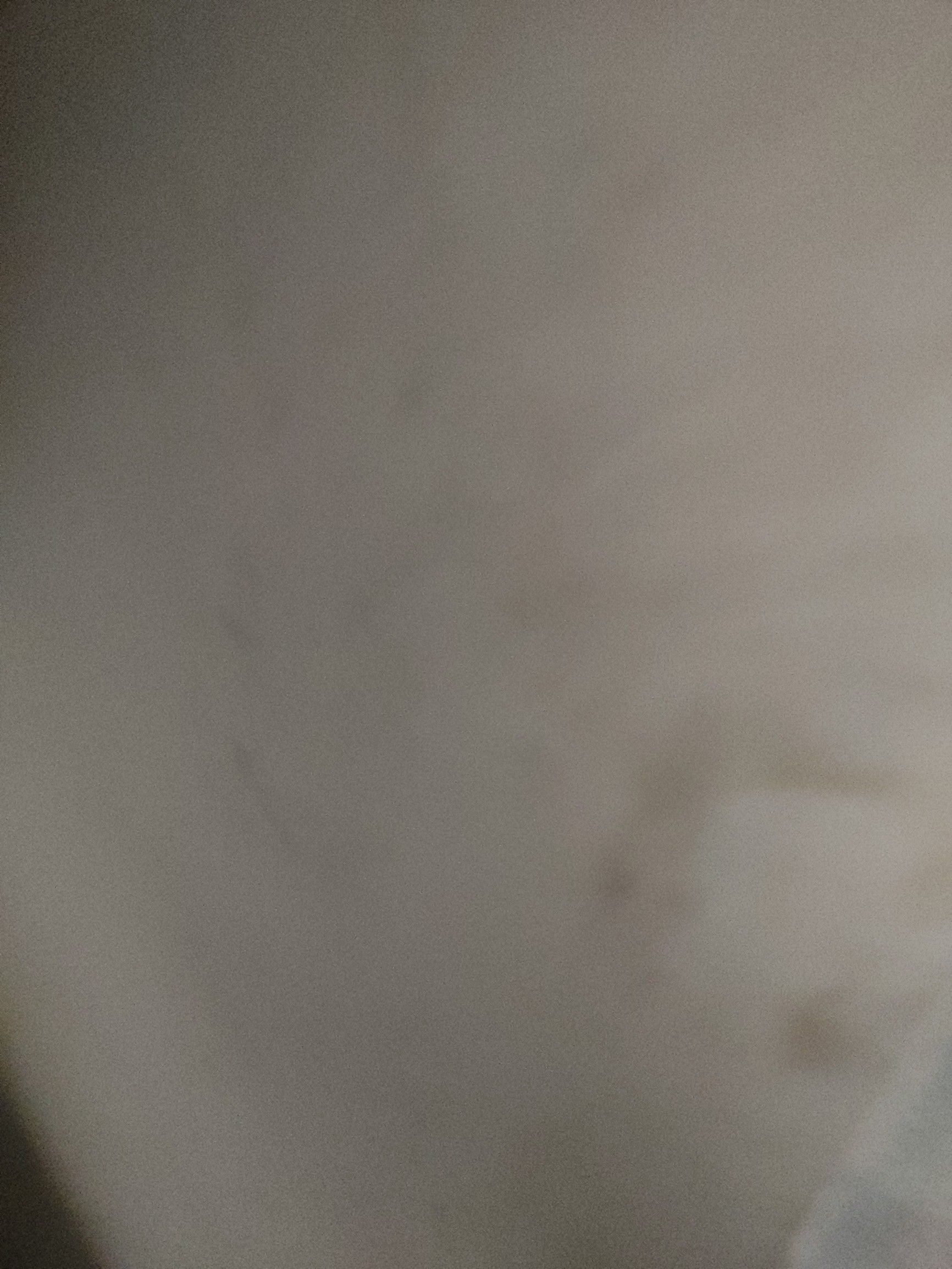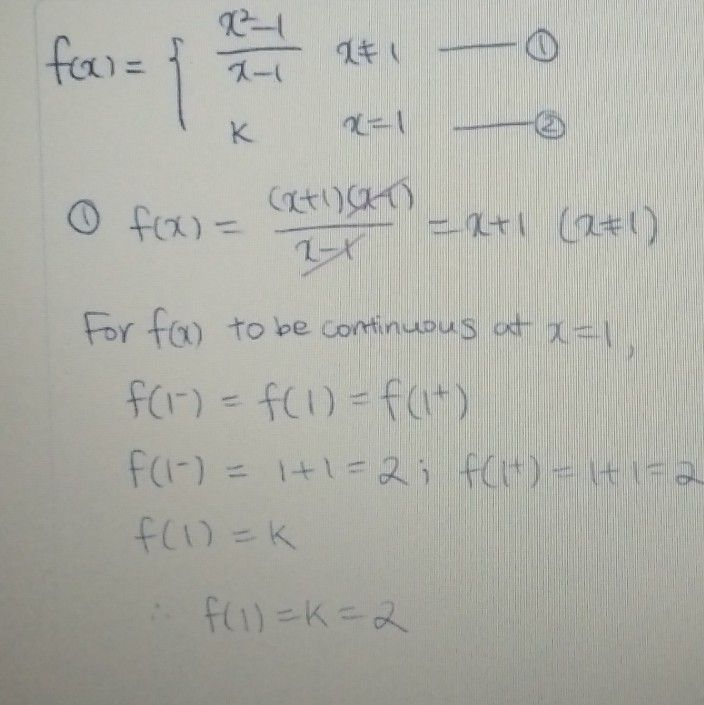Symbol
Problem$3.$ $nf\left(x\right)=\left(\dfrac {x^{2-1}} {x-1}$ $k$ when $x≠1$ If the function when $x=1$ is given to be continuous at $x=1,$ then the value of $k$ is
Physics
SolutionQanda teacher - Kamlesh